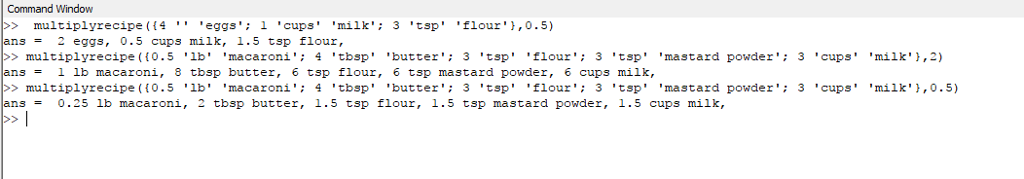# Answered! Using Matlab Write a function that takes a recipe and a coefficient and return a string describing the adjusted recipe. The input…

Using Matlab

Don't use plagiarized sources. Get Your Custom Essay on
Answered! Using Matlab Write a function that takes a recipe and a coefficient and return a string describing the adjusted recipe. The input…
GET AN ESSAY WRITTEN FOR YOU FROM AS LOW AS \$13/PAGE

Write a function that takes a recipe and a coefficient and return a string describing the adjusted recipe. The input recipe is given as a cell array where each row describes one type of ingredient; first column containing a numerical amount, second column containing the units, and third column containing the name of the ingredient. The output should be a string describing the recipe. See the example below. The ingredients should be separated by a comma and space and should end with a period. If an amount is not an integer, show it using 2 decimal places.

```>> multiplyrecipe({4 '' 'eggs'; 1 'cups' 'milk'; 3 'tsp' 'flour'},0.5)

ans =

2  eggs, 0.5 cups milk, 1.50 tsp flour.

>> multiplyrecipe({0.5 'lb' 'macaroni'; 4 'tbsp' 'butter'; 3 'tsp' 'flour'; 3 'tsp' 'mastard powder'; 3 'cups' 'milk'},0.5)

ans =

0.25 lb macaroni, 2 tbsp butter, 1.50 tsp flour, 1.50 tsp mastard powder, 1.50 cups milk.

>> multiplyrecipe({0.5 'lb' 'macaroni'; 4 'tbsp' 'butter'; 3 'tsp' 'flour'; 3 'tsp' 'mastard powder'; 3 'cups' 'milk'},2)

ans =

1 lb macaroni, 8 tbsp butter, 6 tsp flour, 6 tsp mastard powder, 6 cups milk.
```

Please find below the required code:

multiplyrecipe.m

%function will return str after multiplying
%t is cell array and mul is no that will multiply the
%cell array value
function str = multiplyrecipe(t,mul)
%str will return after function done
str=””;
j=0;
for i=1:length(t)
%getting the number from cell array
temp=t(i);
%convering to matrix
A = cell2mat(temp);
%multiplying by given no
A=((A.*mul));
%saving back to cell
t(i)=A;
%converitng to string
C=mat2str(A);
%concating with the string
str=strcat(str,[‘ ‘ C]);
%procesing other cell value
b=t(i,1:3);
for k=2:length(b)
%convering cell cell to matrix
T=cell2mat(b(k));
%concating with the string
str=strcat(str,[‘ ‘ T]);
end
%final string
j=j+1;

if(j<length(t))
str=strcat(str,”, “);
else
str=strcat(str,”.”);

end
end

end %end of function

%OUTPUTPlease do let me know if u have any doubts…